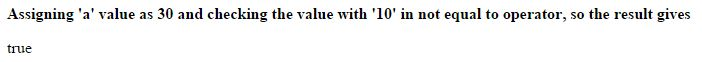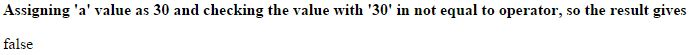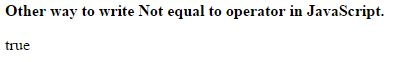# Not equal (!==) operator in JavaScript

##### How to check if the values of two operands are not equal in JavaScript?

In the previous post we learnt the Equal opeartor, from this post we are going to learn the Not equal operator in Comparison Operators.

### Not equal (!==)

Not equal is an comparison operator which is used to check the value of two operands are equal or not.

If the value of two operands are not equal it returns true.

The symbolic representation of Not equal operator in JavaScript is `!=`.

### Assigning different values

```<b>Assigning 'a' value as 30 and checking the value with '10' in not equal to operator, so the result gives</b>
<p id="myId"></p>

<script>
var a = 30;
document.getElementById("myId").innerHTML = (a != 10);
</script>
```

In the above code snippet we have given two different values to the variable `a` and to the not equal operator, so the result gives 'true'.

OUTPUT### Assigning same values

```<b>Assigning 'a' value as 30 and checking the value with '30' in not equal to operator, so the result gives</b>
<p id="myId"></p>

<script>
var a = 30;
document.getElementById("myId").innerHTML = (a != 30);
</script>
```

Not equal opeartor with same values

In the above code snippet we have given same values to the variable `a` and to the not equal operator, so the result give 'false'.

NOTE: The Not equal to operator value can be written as `a != 30` or `a != "30"`, both gives the same result.

OUTPUT### Basic way of using Not equal to operator

```<b>Other way to write Not equal to operator in JavaScript.</b>
<p id="myId"></p>

<script>
var a = 30;
var b = 10;
var c = (a != b);
document.getElementById("myId").innerHTML = c;
</script>
```

In the above code snippet we used the simple way of writing program on Not equal to operator in JavaScript.

OUTPUTViews: 302304 | Post Order: 168